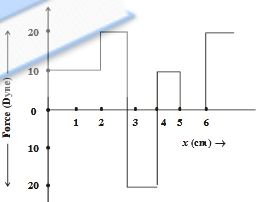1.  How much is the work done in pulling up a block of wood weighing 2KN for alength of 10m on a smooth plane inclined at an angle of $$30^{0}$$ with the horizontal ?

A. 1.732 KJ

B. 17.32 KJ

C. 10 KJ

D. 100 KJ

Explanation :

W=Fd cos\Theta

2.  A force of 7N, making an angle $$Theta$$with the horizantal, acting on an object displacesit by 0.5m along the horizontal direction. If the object gains K.E. of 2J, what is thehorizontal component of the force ?

A. 2 N

B. 4 N

C. 1 N

D. 14 N

Explanation :

$$\Delta K=Fd cos\Theta = W$$

3.  A 60 kg JATAN with 10 kg load on his head climbs 25 steps of 0.20m height each.what is the work done in climbing ? (g = 10 m/$$s^{2}$$)

A. 5 J

B. 350 J

C. 100 J

D. 3500 J

Explanation :

$$W = Fd = (Mg)(d); where M= m_{1}+m_{2}$$

4.  A ball of mass 5 kg is stiding on a plane with intial velocity of 10 m/s. If co-efficient of friction between surface and ball is $$\frac{1}{2}$$, then before stopping it willdescribe...... (g = 10 m/$$s^{2}$$)

A. 12.5 m

B. 5 m

C. 7.5 m

D. 10 m

5.

The relationship between force and position is shown in the figure given (in one dimensional case) calculate the work done by the force in displacing body from x = 0 cm to x = 5 cmA. 30 ergs

B. 70 ergs

C. 20 ergs

D. 60 ergs

6.  The force constant of a wire is K and that of the another wire is 3k when both the wires are stretched through same distance, if work done are $$W_{1}$$and $$W_{2}$$, then..

A. $$w_{2}=3w_{1}^{2}$$

B. $$W_{2}=0.33W_{1}$$

C. $$W_{1}=W_{2}$$

D. $$W_{2}=3W_{1}$$

7.  A ball is released from the top of a tower. what is the ratio of work done by forceof gravity in first, second and third second of the motion of the ball ?

A. 1 : 2 : 3

B. 1 : 4 : 9

C. 1 : 3 : 5

D. 1 : 5 : 3

Explanation :

$$[h_{n} \alpha (2n-1)]$$

8.  A spring of spring constatnt $$10^{3}$$ N/m is stretched initially 4cm from the unstretched position. How much the work required to stretched it further by another5 cm ?

A. 6.5 NM

B. 2.5 NM

C. 3.25 NM

D. 6.75 NM

Explanation :

$$W=\frac{1}{2}k(x_{2}^{2}-X_{1}^2)$$

9.  The mass of a car is 1000 kg. How much work is required to be done on it to make it move with a speed of 36 km/h ?

A. $$2.5\times 10^4 J$$

B. $$5\times 10^3 J$$

C. 500 J

D. $$5\times 10^4 J$$

Explanation :

According to work energy theorm $$W=\Delta k$$

10.  A body of mass 6 kg is under a force, which causes a displacement in it given by $$S=\frac{2t^2}{3}$$ (in m). Find the work done by the force in first one seconds.

A. 2 J

B. 3.8 J

C. 5.2 J

D. 24 J